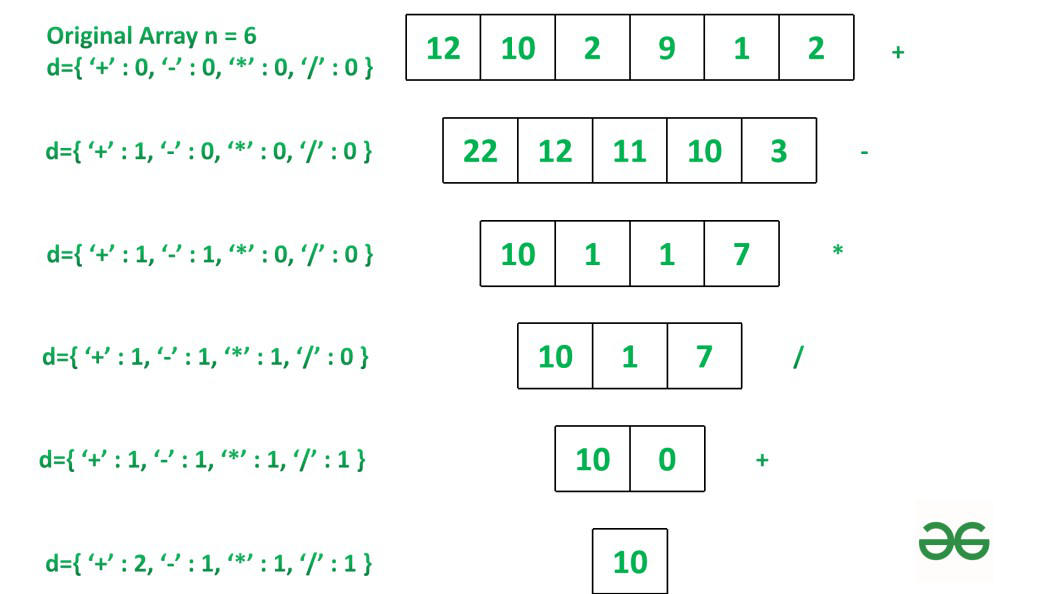Related Articles

# Minimize arithmetic operations to be performed on adjacent elements of given Array to reduce it

• Difficulty Level : Hard
• Last Updated : 10 Oct, 2021

Given an array arr[], the task is to perform arithmetic operations (+, -, *, /) on arr[] in sequence. These operations are performed on adjacent elements until array size reduces to 1. Finally, return the reduced value and number of operations required for the same. Refer to examples for more clarity.

Examples:

Input: arr = {12, 10, 2, 9, 1, 2}
Output: Reduced Value: 10
Operations Performed:
+ : 2
– : 1
* : 1
/ : 1

Explanation:
Step 1: perform addition of consecutive element [22, 12, 11, 10, 3]
Step 2: perform subtraction of consecutive element [10, 1, 1, 7]
Step 3: perform multiplication of consecutive element[10, 1, 7]
Step 4: perform division of consecutive element [10, 0]
Step 5: Again perform addition of consecutive elementInput: arr = {5, -2, -1, 2, 4, 5}
Output: Reduced Value: -3
Operations Performed:
+ : 2
– : 2
* : 1
/ : 1

Approach: Since in this problem we have to perform operations based on the sequence like first addition then subtraction, then multiplication and then division hence we can use Switch case to solve this problem.

Initialize an dictionary where operator (+, -, *, /) as key and 0 as value.  Using functions add, sub, mul and div, operation on array will be performed. It has as function Operation which maps the array with functions based on the value of c%4 and return the reduced array. where c keeps track of number of operations performed. Dictionary keeps track of each operations performed till size of array get reduced to 1.
Follow the steps below to solve given problem.

Step 1: Assign c to 1 and declare dictionary d.

• Step 2: if c%4==1 then perform addition operation on consecutive element using Operation function.

• Step 3: if c%4==2 then perform subtraction operation on consecutive element using Operation function.

• Step 4: if c%4==3 then perform multiplication operation on consecutive element using Operation function.

• Step 5: if c%4==0 then perform division operation on consecutive element using Operation function.

• Step 6: step 2 will be repeated till size of array become equal to 1.

• Step 7: d is used to keep track of each operation performed.

Below is the implementation of the above approach:

## Python3

 `# Function for adding consecutive element``def` `add(a):``    ``return` `[a[i]``+``a[i ``+` `1``] ``for` `i ``in` `range``(``len``(a)``-``1``)]` `# Function for subtracting consecutive element``def` `sub(a):``    ``return` `[a[i] ``-` `a[i ``+` `1``] ``for` `i ``in` `range``(``len``(a)``-` `1``)]` `# Function for multiplication of consecutive element``def` `mul(a):``    ``return` `[a[i] ``*` `a[i ``+` `1``] ``for` `i ``in` `range``(``len``(a) ``-` `1``)]` `# Function for division of consecutive element``def` `div(a):``    ``return` `[``0` `if` `a[i] ``=``=` `0` `or` `a[i ``+` `1``] ``=``=` `0` `\``            ``else` `a[i]``/``/``a[i ``+` `1``] \``                 ``for` `i ``in` `range``(``len``(a) ``-` `1``)]` `# Operation function which maps array``# to corresponding function``# based on value of i.``def` `Operation(i, A):``    ``switcher ``=` `{``        ``1``: add,``        ``2``: sub,``        ``3``: mul,``        ``0``: div``    ``}``    ``func ``=` `switcher.get(i, ``lambda``: ``'Invalid'``)``    ``return` `func(A)`` ` `# Driver Code``c ``=` `1` `# dictionary value which keep track``# of no of operation of each operator.``d ``=` `{``'+'``:``0``, ``'-'``:``0``, ``'*'``:``0``, ``'/'``:``0` `}` `arr ``=``[``5``, ``-``2``, ``-``1``, ``2``, ``1``, ``4``, ``5``]` `# loop till size of array become equal to 1.``while` `len``(arr)!``=` `1``:``    ``x ``=` `c ``%` `4``    ``# After each operation value``    ``# in dictionary value is incremented``    ``# also reduced array``    ``# is assigned to original array.``    ``if` `x ``=``=` `1``:``        ``d[``'+'``] ``+``=` `1``        ``arr ``=` `Operation(x, arr)``    ``elif` `x ``=``=` `2``:``        ``d[``'-'``] ``+``=` `1``        ``arr ``=` `Operation(x, arr)``    ``elif` `x ``=``=` `3``:``        ``d[``'*'``] ``+``=` `1``        ``arr ``=` `Operation(x, arr)``    ``elif` `x ``=``=` `0``:``        ``d[``'/'``] ``+``=` `1``        ``arr ``=` `Operation(x, arr)``    ``c ``+``=` `1` `# Printing reduced value``print``(``"Reduced value:"``, ``*``arr)`  `# Printing value of each operation``# performed to reduce size of array to 1.``print``(``"Operations Performed:"``)``for` `i ``in` `d.keys():``    ``print``(``str``(i) ``+` `" : "` `+``str``(d[i]))`
Output
```Reduced value: -3
Operations Performed:
+ : 2
- : 2
* : 1
/ : 1```

Time Complexity: O(N)
Auxiliary Space: O(N)

Attention reader! Don’t stop learning now. Get hold of all the important mathematical concepts for competitive programming with the Essential Maths for CP Course at a student-friendly price. To complete your preparation from learning a language to DS Algo and many more,  please refer Complete Interview Preparation Course.

My Personal Notes arrow_drop_up